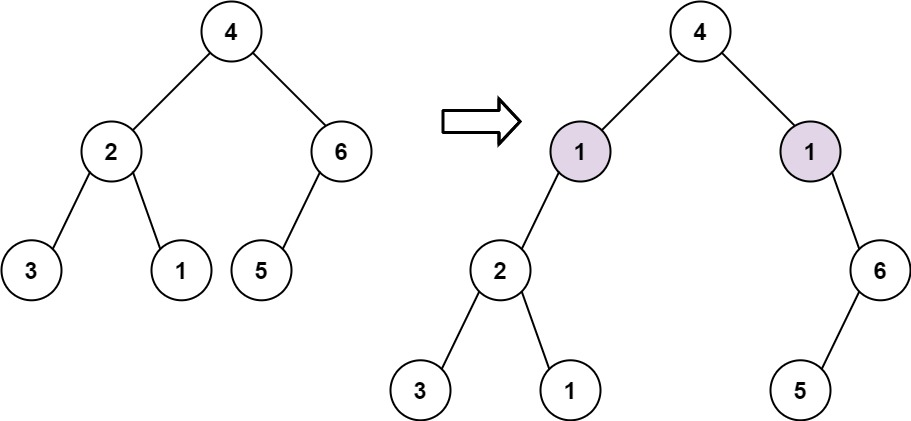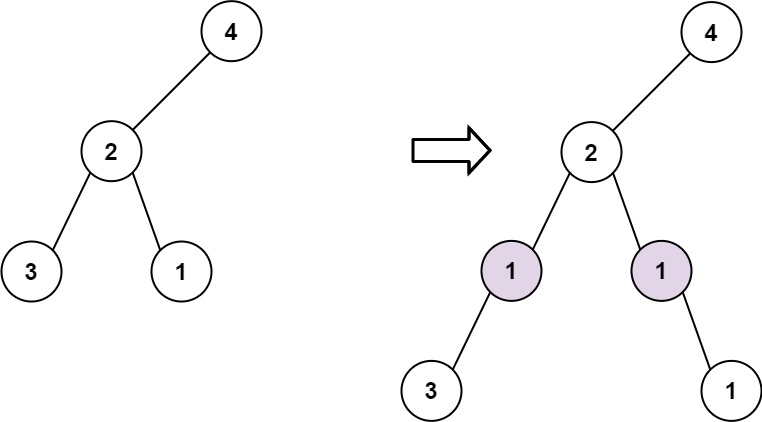# 623. Add One Row to Tree | LeetCode Solution with Explanation

## 623. Add One Row to Tree

Given the `root` of a binary tree and two integers `val` and `depth`, add a row of nodes with value `val` at the given depth `depth`.

Note that the `root` node is at depth `1`.

• Given the integer `depth`, for each not null tree node `cur` at the depth `depth - 1`, create two tree nodes with value `val` as `cur`'s left subtree root and right subtree root.
• `cur`'s original left subtree should be the left subtree of the new left subtree root.
• `cur`'s original right subtree should be the right subtree of the new right subtree root.
• If `depth == 1` that means there is no depth `depth - 1` at all, then create a tree node with value `val` as the new root of the whole original tree, and the original tree is the new root's left subtree.

Example 1:```Input: root = [4,2,6,3,1,5], val = 1, depth = 2
Output: [4,1,1,2,null,null,6,3,1,5]
```

Example 2:```Input: root = [4,2,null,3,1], val = 1, depth = 3
Output: [4,2,null,1,1,3,null,null,1]
```

Constraints:

• The number of nodes in the tree is in the range `[1, 104]`.
• The depth of the tree is in the range `[1, 104]`.
• `-100 <= Node.val <= 100`
• `-105 <= val <= 105`
• `1 <= depth <= the depth of tree + 1`
Solution:
What we will do is we will keep the parent node and whenever we reach our depth we will make a new node that will connect the parent node, the new node, and the current node.

See the Code below for a better understanding.